## On the Subject of 14

Who is high school for? High school is 14agers.

Tn+1 = Tn + Dn Tn+1 = Tn - Dn Tn+1 = Tn + 2Dn Tn = current total for each color Dn = current displayed digit Apply the equation for each separate color. LED Color Digit is normal (> 0) Digit is inverted (< 0) White Black Red Blue Green Magenta Cyan Yellow

Add the value from each color channel to the previous stage’s value.

Note: T0 for R, G, B = indicator label if indicator == 1; otherwise SN1, SN2, SN3.

If Tn > 35, subtract 36 until Tn < 36

If Tn < -35, add 36 until Tn > -36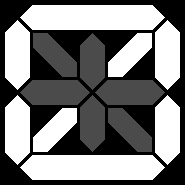0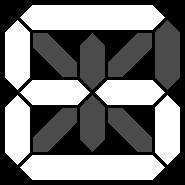6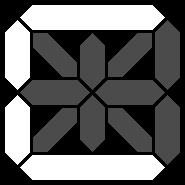C = 12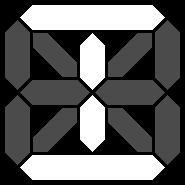I = 18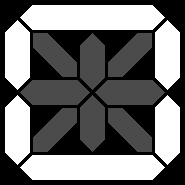O = 24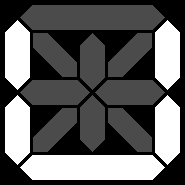U = 30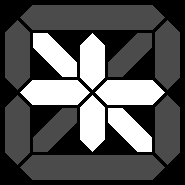-0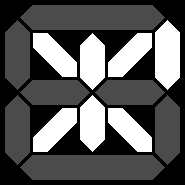-6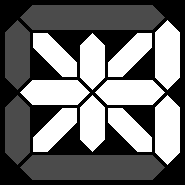-C = -12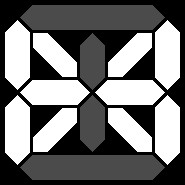-I = -18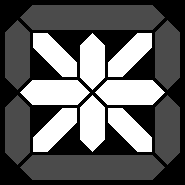-O = -24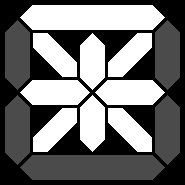-U = -30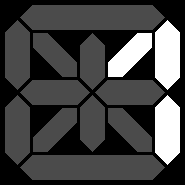1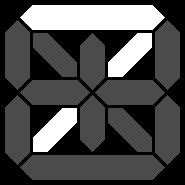7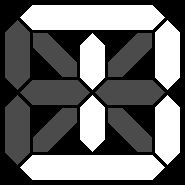D = 13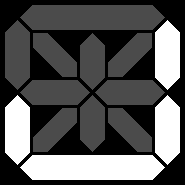J = 19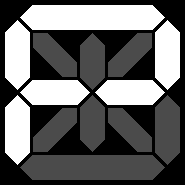P = 25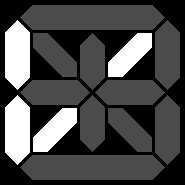V = 31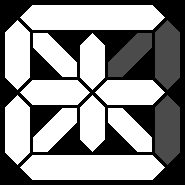-1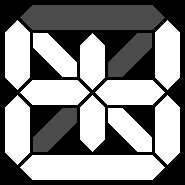-7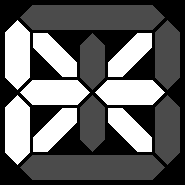-D = -13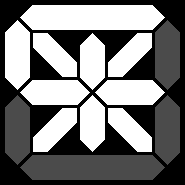-J = -19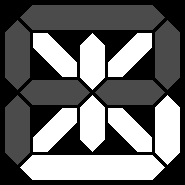-P = -25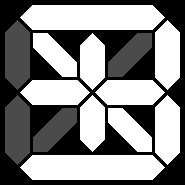-V = -31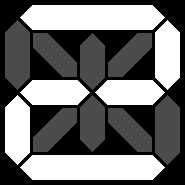2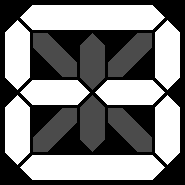8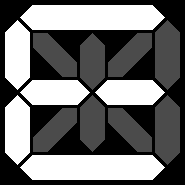E = 14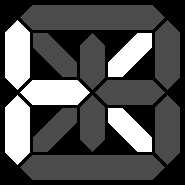K = 20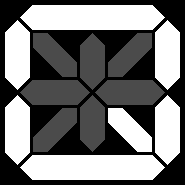Q = 26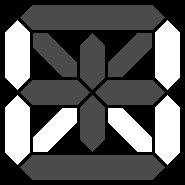W = 32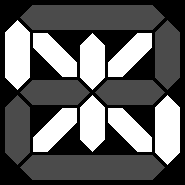-2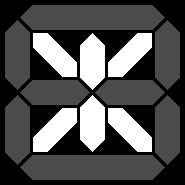-8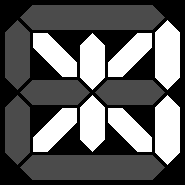-E = -14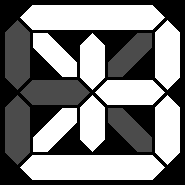-K = -20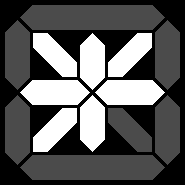-Q = -26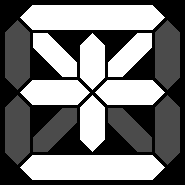-W = -32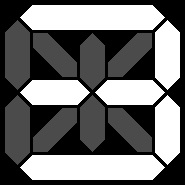3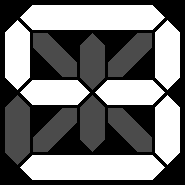9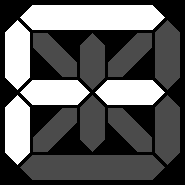F = 15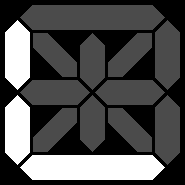L = 21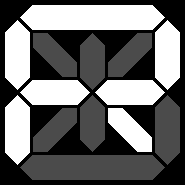R = 27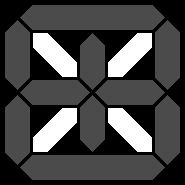X = 33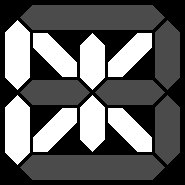-3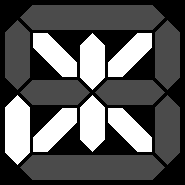-9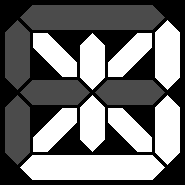-F = -15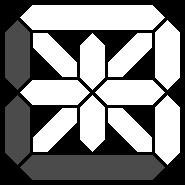-L = -21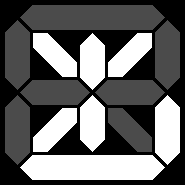-R = -27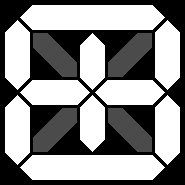-X = -33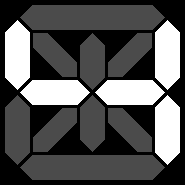4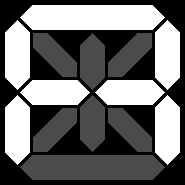A = 10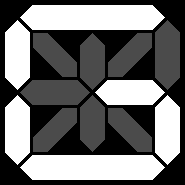G = 16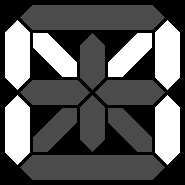M = 22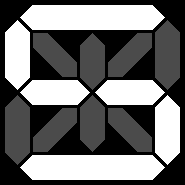S = 28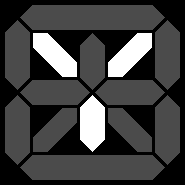Y = 34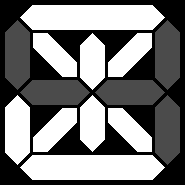-4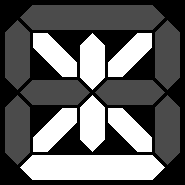-A = -10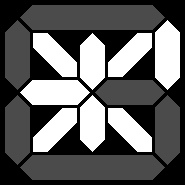-G = -16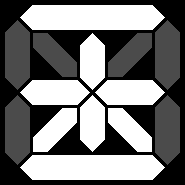-M = -22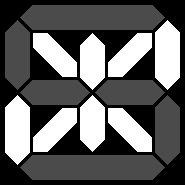-S = -28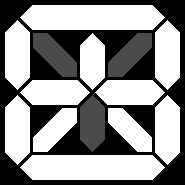-Y = -34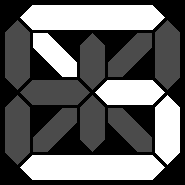5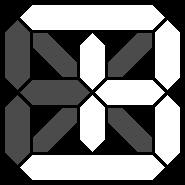B = 11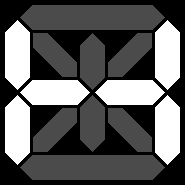H = 17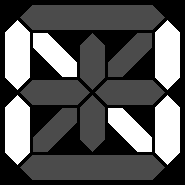N = 23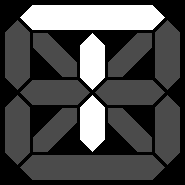T = 29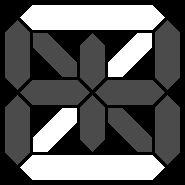Z = 35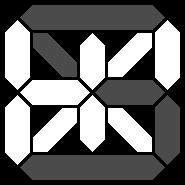-5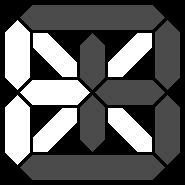-B = -11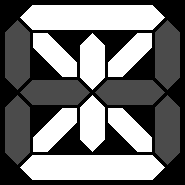-H = -17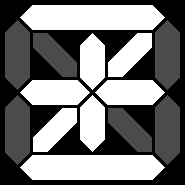-N = -23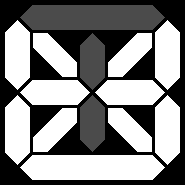-T = -29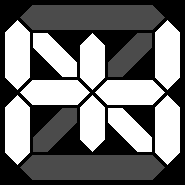-Z = -35